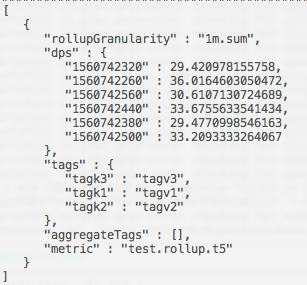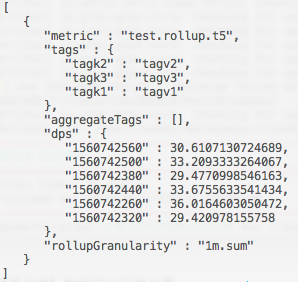All Products
Search
Document Center

# Quick start guide

Last Updated: May 25, 2020

### Rollup example

#### 1. Make preparations for service verification

Create a rollup rule for an instance in the TSDB console.

#### 2. Write data for service verification

This example uses a stress testing application to continuously write a data point every second.

``public static void main(String[] args) throws InterruptedException, IOException {``        //Create a TSDB instance in the staging environment.``     HiTSDBConfig config = HiTSDBConfig.address("{TSDB instance ID}.hitsdb.rds.aliyuncs.com", 3242)``          .listenBatchPut(new BatchPutCallback()``                          {``                            @Override``                            public void response(String s, List<Point> list, Result result) {``                              Point p = Iterables.getFirst(list, null);``                              System.out.println("Put datapoint in second:" + (p == null ? null : p.getTimestamp()));``                            }``                            @Override``                            public void failed(String address, List<Point> request, Exception ex) {``                              System.err.println(ex);``                            }``                          })``          .config();``        HiTSDB tsdb = HiTSDBClientFactory.connect(config);``        //Create data and write the data to TSDB.``        for (int i = 0; i < 3600 * 2; i++) {``            int second = Math.toIntExact(System.currentTimeMillis() / 1000);``            List<Point> points = new ArrayList<Point>();``            points.add(Point``                           .metric("test.rollup.t5")``                           .tag("tagk1", "tagv1")``                           .value(second, Math.random())``                           .build()``            );``            points.add(Point``                           .metric("test.rollup.t5")``                           .tag("tagk1", "tagv1")``                           .tag("tagk2", "tagv2")``                           .value(second, Math.random())``                           .build()``            );``            points.add(Point``                           .metric("test.rollup.t5")``                           .tag("tagk1", "tagv1")``                           .tag("tagk2", "tagv2")``                           .tag("tagk3", "tagv3")``                           .value(second, Math.random())``                           .build()``            );``            tsdb.put(points);``            System.out.println(i);``            Thread.sleep(1000); //Submit data every second.``        }``        //Use a secure method to close the client to prevent data loss.``        System.out.println("Close");``        tsdb.close();``    }``

#### 3. Verify the task status

The task status is continuously updated during the data writing process.

The following three performance metrics are used to check the task status:

• Data points that match the rule within five minutes
• Data points that are to be processed
• Data points in the rollup output within five minutes

#### 4. Verify queries

##### 4.1 Verify queries that are based on raw data

Request (stored as the query.json file)

``{``    "start":1560742264,``    "end":1560742564,``    "queries":[``        {``            "aggregator":"sum",``            "downsample":"1m-sum",``            "metric":"test.rollup.t5",``            "tags":{``                "tagk1":"tagv1",``                "tagk2":"tagv2",``                "tagk3":"tagv3"``            }``        }``    ]``}``

Command

``curl -H "Accept: application/json" -H "Content-type: application/json" -X POST --data @query.json http://Instance IP address:3242/api/query``

Result ([raw data])##### 4.2 Verify queries that are based on rollups

Request (stored as the query_rollup.json file)

``{``    "start":1560742264,``    "end":1560742564,``    "queries":[``        {``            "aggregator":"avg",``            "downsample":"1m-sum",``            "metric":"test.rollup.t5",``            "tags":{``                "tagk1":"tagv1",``                "tagk2":"tagv2",``                "tagk3":"tagv3",``                "__granularity__":"1m.sum"``            }``        }``    ]``}``

Command

``curl -H "Accept: application/json" -H "Content-type: application/json" -X POST --data @query_rollup.json http://Instance IP address:3242/api/query``

Result ([rollups])Note that the query result contains the rollupGranularity tag. This indicates that rollups are used for this query. In the query result, you can also find the operator that is used for scanning data points.